# Extracting Point Information from Hangers in CADMEP+

## Recommended PostsDoes anyone have any knowledge on how to extract the point information from a CADMEP entity without having the CADMEP information?

Our clients give us there files and we have to place blocks at each hanger. Extracting the coordinates to populate the blocks would be a huge time saver.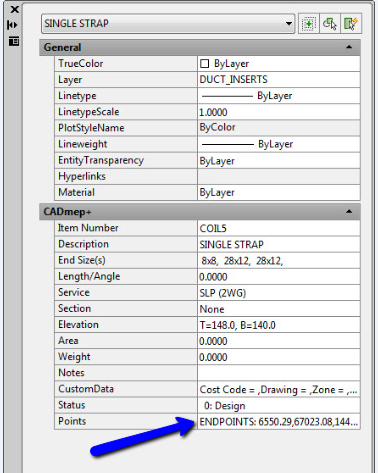##### Share on other sites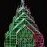This topic was discussed in another forum here:

http://www.theswamp.org/index.php?topic=45166.0

Maybe this will help...

##### Share on other sitesThis topic was discussed in another forum here:

http://www.theswamp.org/index.php?topic=45166.0

Maybe this will help...

I forgot to say thank you LibertyOne!

##### Share on other sites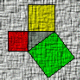TheyCallMeJohn,

Since you are getting this listing, "points" is very likely to be a property of a hanger object.

To confirm issue:

`(vlax-dump-object (vlax-ename->vla-object (car (entsel "\nSelect Object:"))) t)`

If it is a property, then you can access it with:

```(vlax-get-property (vlax-ename->vla-object (car (entsel "\nSelect Object:"))) 'points)
```

The above assume that points is the property name. You would then need to parse

whatever you get as a return.

ymg

##### Share on other sitesSo I figured a way to pull out the points, kind of what you recommended Ymg3. It seems to work if I copy and paste the code in line by line but when I try and run it on a group of items. It goes to the wrong points. I uploaded an SWF and dwg here. To show what is happening.

```	(setq pl_set (ssget '((0 . "MAPS_SOLID"))))
(setq pl_set_lgth (SSLENGTH pl_set))
(setq pl_item 0)
(repeat pl_set_lgth
(setq en (SSNAME pl_set pl_item))
(setq entvla (vlax-Ename->Vla-Object en))
(setq unitdecrp (vlax-get entvla 'description))
(if (vl-string-search "hanger" unitdecrp)
(progn
(setq unitpoint (vl-string-right-trim ";" (substr (vlax-get entvla 'points) 12)))
(command "insert" pl_block unitpoint "1" "1" "0")
)
(princ "\n Invalid Objects")
)
(setq pl_item (+ pl_item 1))
)	```

##### Share on other sitesTheyCallMeJohn,

But from the symbols there seems to be two different type of hangers.

ymg

##### Share on other sitesI was experimenting around and it worked after I changed this....

```(if (vl-string-search "hanger" unitdecrp)
```

To this:

```(if (/= (vl-string-search "hanger" unitdecrp) nil)
```

Go figure...

Oh and Ymg I am stuck with Vanillia Autocad but I am supposed to figure out how to pull information out of the CadDuct database... *bangs head against wall*

Edited by TheyCallMeJohn
Forgot something....

##### Share on other sitesTheyCallMeJohn,

Those two are normally equivalent. What are we missing ? So maybe we should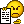ymg

##### Share on other sitesI thought so too but I thougth what the hell, I generally don't know what I am doing, I will give it try! (...not really expecting it it to work). yet alas it did work.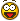Wait there is a manual?!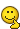##### Share on other sitesSo I am utilizing some commands that new to me and I have run into a kind of a weird issue. I am utilizing a bounding box determine the orientation of the MAP_SOLIDS duct straps but it seem to take the orientation of the first and not check the orientation of the rest. That or made a mistake in my code.... (very likely). Any suggestion?

```((= hngr_trgt_obj "MAPS_SOLID")
(progn
(princ "\n This is MAP Solid. Selected layer will be ignored.\N Select hangers to populate:  ")
(setq pl_set (ssget '((0 . "MAPS_SOLID"))))
(setq pl_set_lgth (SSLENGTH pl_set))
(setq pl_item 0)
(repeat pl_set_lgth
(setq en (SSNAME pl_set pl_item))
(setq entvla (vlax-Ename->Vla-Object en))
(setq unitdecrp (vlax-get entvla 'description))
(cond
((or (/= (vl-string-search "hanger" unitdecrp) nil)(and (= (vl-string-search "Hanger Strap" unitdecrp) nil) (/= (vl-string-search "Hanger" unitdecrp) nil))(/= (vl-string-search "SINGLE STRAP" unitdecrp) nil))
(progn
(setq unitpoint_orig (vl-string-right-trim ";" (substr (vlax-get entvla 'points) 12)))
(if (and(or (= Z_pref "Y")(= Z_pref "y"))(/= Z_height nil))
(progn
(setq unitpoint_mod (comma_pull unitpoint_orig "REAL_NUM"))
)
(setq unitpoint (comma_pull unitpoint_orig "REAL_NUM"))
)
(setq unitpoint_lst (append unitpoint_lst (list unitpoint)))

)
)
((or (/= (vl-string-search "Hanger Strap" unitdecrp) nil)(/= (vl-string-search "Strap" unitdecrp) nil)(/= (vl-string-search "STRAP" unitdecrp) nil))
(progn
(setq unitpoint_orig (vl-string-right-trim ";" (substr (vlax-get entvla 'points) 12)))
(if (and(or (= Z_pref "Y")(= Z_pref "y"))(/= Z_height nil))
(progn
(setq unitpoint_mod (comma_pull unitpoint_orig "REAL_NUM"))
)
(setq unitpoint (comma_pull unitpoint_orig "REAL_NUM"))
)
(setq unit_endsizes (vlax-get entvla 'endsize))
(setq x_pos (vl-string-search "x" unit_endsizes))
(setq duct_width (atoi (substr unit_endsizes 1 x_pos)))
(vla-GetBoundingBox entvla 'minpoint 'maxpoint)
(setq minpointlist (vlax-safearray->list minpoint))
(setq x_coord_min (car minpointlist))
(setq maxpointlist (vlax-safearray->list maxpoint))
(setq x_coord_max (car maxpointlist))
(setq pnt_angle (RTD (angle (list x_coord_min y_coord_min)(list x_coord_max y_coord_max))))
(setq pnt_angle_R (angle (list x_coord_min y_coord_min)(list x_coord_max y_coord_max)))
(cond
((and (>= pnt_angle 40.0)(<= pnt_angle 50.0))
(progn
(setq delta_x (* (cos pnt_angle_R) (/ duct_width 2)))
(setq delta_y (* (sin pnt_angle_R) (/ duct_width 2)))
(setq unitpoint_1 (list (+ (car unitpoint) delta_x) (+ (cadr unitpoint) delta_y) (caddr unitpoint)))
(setq unitpoint_2 (list (- (car unitpoint) delta_x) (- (cadr unitpoint) delta_y) (caddr unitpoint)))
)
)
((> pnt_angle 50.0)
(progn
(setq unitpoint_1 (list (car unitpoint) (+ (cadr unitpoint) (/ duct_width 2)) (caddr unitpoint)))
(setq unitpoint_2 (list (car unitpoint) (- (cadr unitpoint) (/ duct_width 2)) (caddr unitpoint)))
)
)
((< pnt_angle 50.0)
(progn
(setq unitpoint_1 (list (+ (car unitpoint) (/ duct_width 2)) (cadr unitpoint) (caddr unitpoint)))
(setq unitpoint_2 (list (- (car unitpoint) (/ duct_width 2)) (cadr unitpoint) (caddr unitpoint)))
)
)
)
(setq unitpoint_lst (append unitpoint_lst (list unitpoint_1)))
(setq unitpoint_lst (append unitpoint_lst (list unitpoint_2)))
)
)
(t (princ "\n Invalid Objects"))
)
(setq pl_item (+ pl_item 1))
)
)
)```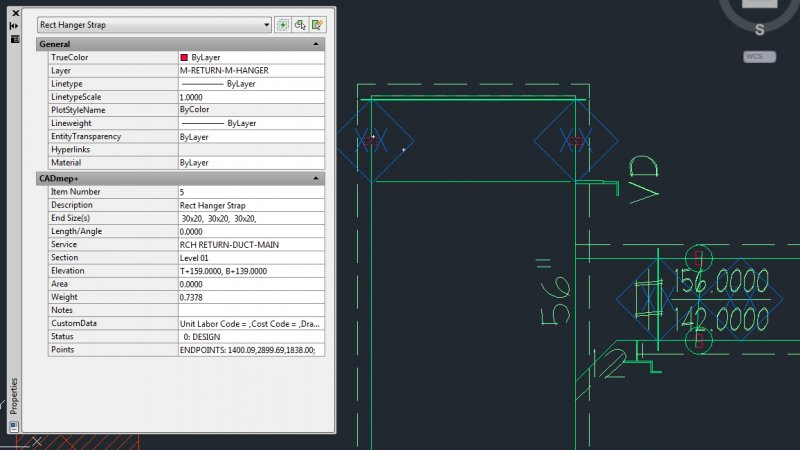Edited by TheyCallMeJohn

## Join the conversation

You can post now and register later. If you have an account, sign in now to post with your account.
Note: Your post will require moderator approval before it will be visible.×   Pasted as rich text.   Paste as plain text instead

Only 75 emoji are allowed.